# Finite Element Methods (FEM)## Finite Element Methods for Members Parallel to the Global X-Axis

The finite element method is one of popular modern methods used for the analysis of structural members. The following statements are also true about the Finite Element Method:

~ Most popular method so far in structural mechanics developed since the 1960’s in parallel by:

§ CIVIL ENGINEERS developed the DIRECT STIFFNESS METHOD

§ MATHEMATICIANS developed the variational principle, and the principle of virtual work.

~ The direct stiffness method is used for sparse structures like trusses and beams.

Key concepts in finite element methods include: element stiffness matrix, global stiffness matrix, assembly, support (boundary) conditions.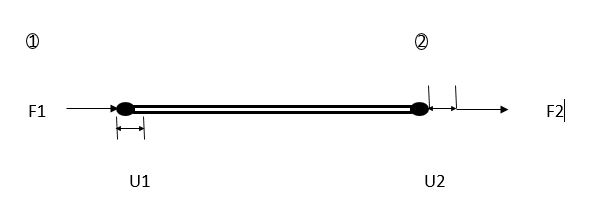Where:

F1 = Force pushing into bar at node 1 and causing compression of bar; hence, let us designate it as a compressional force with a negative sign. This however, will be resisted by an equal and opposite reaction N.

F2 = Force pulling out of bar at node 2 and causing tension in the bar; hence, let us designate it as a tensile force with a positive sign. This will be resisted by an equal and opposite reaction.

That is:

F1 = N

F2 = -N

We know from elementary principles that:

Stress σ = F/A

Where: F = force, and A = Area

Also:

Strain Ɛ = ∆L/L

Where:

∆L = change in length, and L= original length.

But young modulus E = stress/strain.

Therefore,

E = (F/A) / (∆L/L).

E = F/A * L/∆L

E = FL/A∆L

EA∆L/L = F = EA(U2-U1)/L

F1 = -EA(U1-U2)/L

F2 = EA(U1-U2)/LThe above solution is only applicable to bars that are parallel to the global x – axis.

## Finite Element Methods for Inclined Members

F1 = F1cosθ / F1sinθ = F1x / F1y

F2 = F2cosθ / F2sinθ = F2x / F2yHence,Where c = cosθ,

s = sinθ.

Similarly for the displacements,Multiply both sides by the transpose of the transformation matrix ([{T^t}])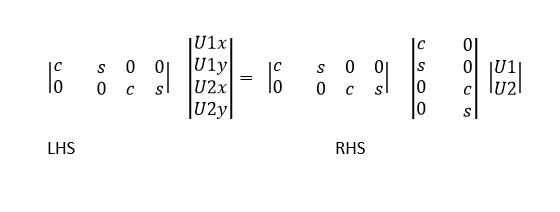Considering the right hand sideRecall from trig. Identities that [co{s^2}theta {rm{ }} + {rm{ }}si{n^2}theta {rm{ }} = {rm{ }}1]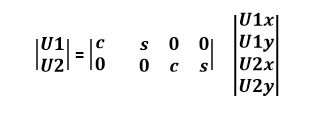From equation 2,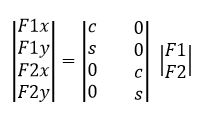Recall that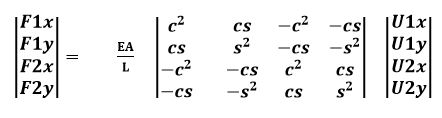This is the elemental force-displacement rotation for a truss member. This holds true for one truss member.

Originally posted on January 24, 2020 @ 10:14 pm